Computer Science‎ > ‎

### Python for Data: (1) Baby step with python for Data Science (word count)

Okay folks, we are going to start gentle with a baby step in python for data Science. We will make a simple program called word count. Linux user probably know this as the wc utility. On Linux, you can type: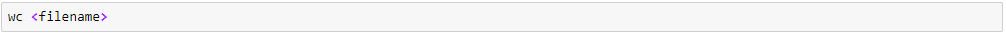I am well aware that it's a piece of cake for many of you out there but to support and bring everyone on the same level of understanding, it's crucial to start from this baby step.

Let's look at python code for counting word in any given file. The very first step would be to import any library or dependencies if required. Here we are gonna use a library called "Collections"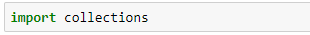Now we can use this library to perform word count and also to save our word statistics as a Excel sheet. We simply open a file called “small.txt”. It must exist in the current directory (ie, the directory you are running the code from). In apposite case you need to enter the whole directory path not just file name.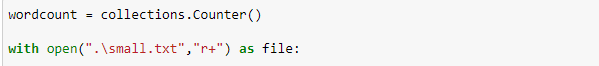Python has several in built functions for strings. One is the split() function which splits the string on the given parameter. In the example above, we are splitting on a space. The function returns a list (which is what Python calls arrays) of the string split on space. In Natural language processing (NLP) people call it 'Tokens'

To see how this works, I’ll fire up an  Anaconda jupyter console.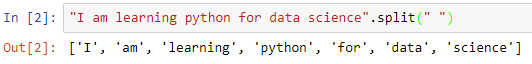Now since we have witnessed how 'split()' built in function works, let's come back to our target.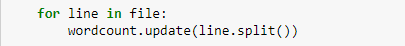This 'for loop' will split every word from each line in our given file.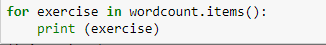Here we done with the counting word from a text file. The output of the above script is as follows -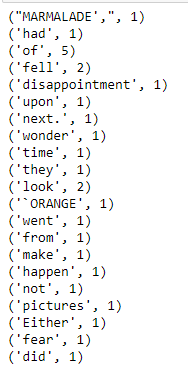This is partial output of our program. Now let's take it to a step further and save the statistics in an Excel file but here we need to import one more dependency called 'xlsxwriter'. Moreover, in python we can save every stats or graphs by writing single line of code unlike other programming languages.Now creating a workbook and saving it in a specific directory where we want and creating a worksheet in the workbook.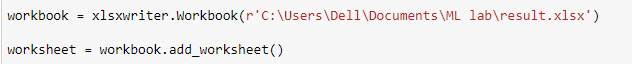Now initialising the rows and coloumns to zero since they start with zero in spreadsheet and iterating through words and writing them in a spreadsheet.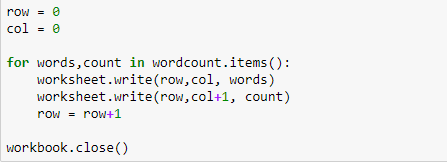Now we can find our word counts in the directory specified above as an Excel file. Let's look at it.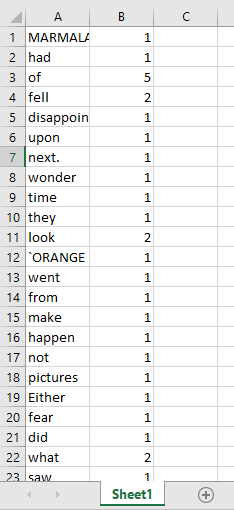Here we finished the baby step of learning Python for data science.

Whats' Next?
Matrix operations
Regression algorithm